# HBSE 9th Class Science Solutions Chapter 3 Atoms and Molecules

Haryana State Board HBSE 9th Class Science Solutions Chapter 3 Atoms and Molecules Textbook Exercise Questions and Answers.

## Haryana Board 9th Class Science Solutions Chapter 3 Atoms and Molecules

HBSE 9th Class Science Atoms and Molecules Intext Questions and Answers

Questions from Sub-section 3.1

Question 1.
In a reaction, 5.3 g sodium carbonate reacted with 6.0 g of ethanoic acid. The products were 2.2 g of carbon dioxide. 0.9 g water and 8.2 g of sodium ethanoate. Show that these observations are in agreement with the law of conservation of mass. Sodium carbonate + ethanoic acid → sodium ethanoate + carbon dioxide + water
Solution:
Here,
Total mass of reactants = Mass of (Sodium carbonate + Ethanoic acid) = 5.3g + 6.0g = 11.3 g
Total mass of products = Mass of(sodium ethanoate + carbon dioxide + water) = 2.2g + 8.2g + 0.9 g = 11.3g
Since, the total mass of reactants is equal to that of the total mass of products, hence these observations are the law of conservation of mass.

Question 2.
Hydrogen and oxygen combine in the ratio of 1:8 by mass to form water. What mass of oxygen gas would be required to react completely with 3 g of hydrogen gas?
Solution:
Here,
Mass of hydrogen:
mass of oxygen = 1:8
Therefore, the required mass of oxygen to combine it completely with 3g of hydrogen = 3g x 8 = 24 gQuestion 3.
Which postulate of Dalton’s atomic theory is the result of the law of conservation of mass?
The postulate of Dalton’s atomic theory is that atoms are the smallest and indivisible particles which can neither be created nor destroyed in chemical reactions. It is the result of the law of conservation of mass.

Question 4.
Which postulate of Dalton’s atomic theory can explain the law of definite proportions?
The postulate which states that atoms of the same or different elements combine in the ratio of small whole numbers to form compounds can explain the law of definite proportions.

Questions from Sub-section 3.2

Question 1.
Define the atomic mass unit.
1/12th part of the mass of one atom of carbon-12 isotope is taken to be the standard atomic mass unit. With respect to the mass of one atom of carbon-12 isotope atomic masses of all the elements have been obtained.

Question 2.
Why is it not possible to see an atom with naked eyes?
Being very small in size, atom cannot be seen with naked eye. Its size is so small that its radius is measured in nanometre (nm). Where, nm = 10 9m. Now, with the help of modem technique, the magnified images of surfaces of elements can be displayed, in which the existing atoms are clearly visible.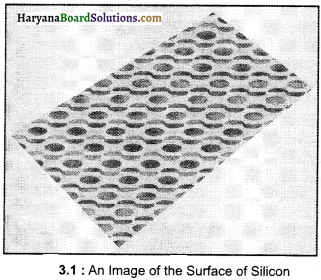Questions from Sub-section 3.4

Question 1.
Write down the formulae of:
(i) sodium oxide
(ii) aluminium chloride
(iii) sodium sulphide
(iv) magnesium hydroxide.
(i) Formula of sodium oxide Symbol Na2O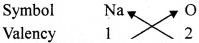∴ The formula of sodium oxide is Na2O.

(ii) Formula of aluminium chloride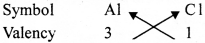∴ The formula of aluminium chloride is AlCl3

(iii) Formula of sodium sulphide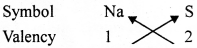∴ The formula of sodium sulphide is Na2S.

(iv) Formula of magnesium hydroxide Symbol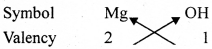∴ The formula of magnesium hydroxide is Mg (OH)2.Question 2.
Write down the names of compounds represented by the following formulae :
(i) Al2(SO4)3
(ii) CaCl2
(iii) K2SO4
(iv) KNO3
(v) CaCO3

 Chemical formula Name of compound (i) Al2 (SO4)3 Aluminium Sulphate (ii) CaCl2 Calcium Chloride (iii) K2 SO4 Potassium Sulphate (iv) KNO3 Potassium Nitrate (v) CaCO3 Calcium Carbonate

Question 3.
What is meant by the term chemical formula?
The chemical formula of a compound is the symbolic representation of its composition,

Question 4.
How many atoms are present in a
(i) H2S molecule
(ii) PO43-ion?
(i) Number of atoms in H2S = 2 + 1 = 3
(ii) Number of atoms in PO3-4 ion = 1 + 4 = 5

Questions from Sub-section 3.5

Question 1.
Calculate the molecular masses of H2, O2, Cl2, CO2, CH4, C2H6, C2H4, NH3, and CH3OH.
Solution:
(1) Atomic mass of hydrogen = lu
H2 contains two atoms of hydrogen.
Molecular mass of H2 = 2 × 1 = 2u

(2) Atomic mass of oxygen = 16u
O2 consists of two atoms of oxygen.
The molecular mass of O2

(3) Atomic mass of chlorine Cl2 consists of two atoms of chlorine.
The molecular mass of Cl2

(4) Atomic mass of carbon = 12u
The atomic mass of oxygen = 16u
.’. CO2 in which there is one atom of carbon and two atoms of oxygen.
Molecular mass of CO2 =1 × 12 + 2 × 16= 12 + 32 = 44u(5) Atomic mass of carbon = 12u
The atomic mass of hydrogen = lu
CH4 where one atom of carbon and four atoms of hydrogen are there.
Molecular mass of CH4 = 1 × 12 + 4 × 1 = 12 + 4 = 16u

(6) Atomic mass of carbon = 12u
The atomic mass of hydrogen = lu
C2H6 in which two atoms of carbon and six atoms of hydrogen are there.
Molecular mass of C2H6 = 2 × 12 + 6 × 1 = 24 + 6 = 30u

(7) Atomic mass of carbon = 12u
The atomic mass of hydrogen = 1u
C2H4 in which two atoms of carbon and four atoms of hydrogen are there.
Molecular mass of C2H4 = 2 × 12 + 4 × 1 = 24 + 4 = 28u

(8) Atomic mass of nitrogen = 14u.
The atomic mass of hydrogen = 1u
.’. NH3 in which one atom of nitrogen and three atoms of hydrogen are there.
Molecular mass of NH3 =1 × 14 + 3 × 1 = 14 + 3 = 17u

(9) Atomic mass of carbon = 12u
The atomic mass of hydrogen = 1u
The atomic mass of oxygen = 16u
CH3OH, in which one atom of carbon, four atoms of hydrogen and one atom of oxygen are there.
Molecular mass of CH3OH = 1 × 12 + 4 × 1 + 16 × 1 = 12 + 4 + 16 = 32u

Question 2.
Calculate the formula unit masses of ZnO, Na2O, K2CO3, given atomic masses of Zn = 65 u, Na = 23 u, K = 39 u, C = 12 u, and O = 16 u.
(1) Formula unit mass of ZnO = 1 × 65u + 1 × 16u = 65u + 16u = 81u
(2) Formula unit mass of Na2O = 2 × 23u + 1 × 16u = 46u + 16u = 62u
(3) Formula unit mass of K2CO3 = 2 × 39u + 1 x 12u + 3 × 16u = 78u + 12u + 48u = 138u

Questions from Sub-section 3.5.3

Question 1.
If one mole of carbon atoms weighs 12 grams, what is the mass (in gram) of 1 atom of carbon 7
Solution:
Here,
Molar mass of carbon = 12g
1 mole = 6.022 x 1023atom
That is, mass of 6.022 x 1023 carbon atoms = 12 g
Mass of 1 carbon atom = $$\frac{12}{6.022 \times 10^{23}}$$ g
= 1.993 x 10-23g

Question 2.
Which has more atoms, 100 grams of sodium or 100 grams of iron (given, the atomic mass of Na = 23 u, Fe = 56u) ?
Solution:
The molar mass of sodium = 23g
1 mole = 6.022 x 1023 atoms
Numbers of atoms in 23g of Na = 6.022 x 1023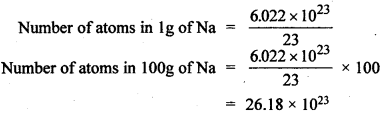Molar mass of iron = 56g
1 mole = 6.022 x 1023
Numbers of atoms in 56g of Na = 6.022 x 1023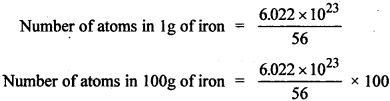= 10.75 × 1023
Thus, there will be more atoms in 100 g of sodium as compared to 100 g of iron.HBSE 9th Class Science Atoms and Molecules Textbook Questions and Answers

Question 1.
A 0.24 g sample of a compound of oxygen and boron was found by analysis to contain 0.096 g of boron and 0.144 g of oxygen. Calculate the percentage composition of the compound by weight.
Solution:
Quantity of boron in 0.24g compound = 0.096 g
Quantity of boron in 1g compound = $$\frac{0.096}{0.24}$$
Quantity of boron in 100g compound = $$\frac{0.096}{0.24} \times 100$$ = 40g
Therefore, the quantity of boron in the compound = 40%
Quantity of oxygen in 0.24 g compound = 0.144g
Quantity of oxygen in 0.24 g compound = $$\frac{0.144}{0.24} \times 100$$ = 60g
Therefore, the quantity of oxygen in the compound = 60%

Question 2.
When 3.0 g of carbon is burnt in 8.00 g of oxygen, 11.00 g of carbon dioxide is produced. What mass of carbon dioxide will be formed when 3.00 g of carbon is burnt in 50.00 g of oxygen? Which law of chemical combination will govern your answer?
3.0g carbon when burnt in 8.00g oxygen produces 11.00 g carbon dioxide, then on burning 3.00 g carbon in 50.00 g oxygen, 53.00 g carbon dioxide will be formed which is based on the law of conservation of mass of chemical combination.Question 3.
What are polyatomic ions? Give examples.
A group of atoms that acts as ions are called as polyatomic ions. For example:

 Polyatomic ions Symbol ammonium NH+4 hydroxide OH– nitrate NO–3 hydrogen carbonate HCO–3 carbonate CO2-3 sulphate SO2-4 phosphate PO3-4

Question 4.
Write the chemical formulae of the following:
(a) Magnesium chloride
(b) Calcium oxide
(c) Copper nitrate
(d) Aluminium chloride
(e) Calcium carbonate.
(a) Formula of magnesium chloride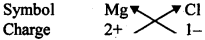∴ Formula of magnesium chloride = MgCl2

(b) Formula of calcium chloride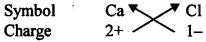∴ The formula of calcium chloride = CaCl2

(c) Formula of copper nitrate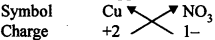∴ The formula of copper nitrate = Cu (NO3)2

(d) Formula of aluminium chloride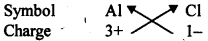∴ Formula of aluminium chloride = AlCl3

(e) Formula of Calcium Carbonate chloride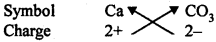∴ The formula of calcium carbonate = CaCO3Question 5.
Give the names of the elements present in the following compounds :
(a) Quick lime
(b) Hydrogen bromide
(c) Baking powder
(d) Potassium sulphate
(a) Quick lime = Ca (OH)2
Thus, in quick lime, the present elements are calcium (Ca), oxygen (O) and hydrogen (H).

(b) Hydrogen hromide = HBr
Thus, in hydrogen bromide, the present elements are hydrogen (H) and bromine (Br).

(c) Baking powder = NaHCO3
Thus, in baking powder, the present elements are sodium (Na), hydrogen (H), carbon (C) and oxygen (O).

(d) Potassium sulphate = K2SO4
Thus, in potassium sulphate, the present elements are potassium (K), sulphur (S) and oxygen (O).

Question 6.
Calculate the molar mass of the following substances:
(a) Ethyne, C2H2
(b) Sulphur molecule, S8
(c) Phosphorus molecule, P4 (Atomic mass of phosphorus = 31)
(d) Hydrochloric acid, HCl
(e) Nitric acid, HNO3
Solution:
We know that C = 12, H = 1, S = 32, P = 31, Cl = 35.5, N = 14, O = 16
(a) Molar mass of ethyne (C2H2) = 2 × 12 + 2 × 1 = 24 + 2 = 26g
(b) Molar mass of sulphur molecule (S8) = 8 × 32 = 256g
(c) Molar mass of phosphorus molecule (P4) = 4 × 31 = 124g
(d) Hydrochloric acid, HCl = 1 × 1 + 1 × 35.5 = 1 + 35.5 = 36.5g
(e) Nitric acid, HNO3 = 1 × 1 + 1 × 14 + 3 × 16= 1 + 14 + 48 = 63gQuestion 7.
What Is the mass of
(a) 1 mole of nitrogen atoms?
(b) 4 moles aluminium atoms (Atomic mass of aluminium = 27)?
(c) 10 moles sodium suiphite (Na2SO3)?
Solution:
(a) Atomic mass of 1 mole of nitrogen = 14 g
(b) Atomic mass of 1 mole of aluminium = 27 g
Atomic mass of 4 moIes of aluminium = 4 × 27 = 108 g
(c) Atomic mass of 1 mole of sodium suiphite (Na2SO3) = 2 × 23 + 1 × 32 + 3 ×16 = 46 + 32 + 48 = 126 g
Atomic mass of 10 moles of sodium sulphite (Na2SO3) = 10 × 126 g = 1260 g

Question 8.
Convert Into mole.
(a) 12 g oxygen gas
(b) 20 g water
(c) 22 g carbon dioxide
Solution:
(a) We know that 1 mole oxygen(O2) 2 × 16 = 32 g
Therefore, 32g oxygen = 1 mole
1g oxygen = $$\frac {1}{2}$$ mole
12g oxygen = $$\frac {1}{2}$$ × 12 = 0.375 mole

(b) We know that l mole water (H2O) = (2 × 1 + 1 × 16)g = (2 +1 6)g = 18g
Therefore, 18g water = 1 mole
1g water = $$\frac {1}{18}$$ mole
20 gwater = $$\frac {1}{18}$$ × 20 = 1.11 mole

(c) We know that I mole carbon dioxide (CO2) = (1 × 12 + 2 × 16)g= (12 + 32)g = 44g
Therefore, 44g carbon dioxide = 1 mole
1g carbon dioxide = $$\frac {1}{44}$$ mole
22g carbon dioxide = $$\frac {1}{44}$$ × 22 = 0.5 mole

Question 9.
What is the mass of
(a) 0.2 mole of oxygen atoms?
(b) 0.5 mole of water molecules?
Solution:
(a) I mole of oxygen atoms = 16g
0.2 mole of oxygen atoms 16 × 0.2g = 3.2g
(b) 1 mole of water molecules (H2O) (2 × 1 + 1 × 16)g = (2 + 16) g = 18g
0.5 mole of water molecules = 0.5 × 18 = 9.0g

Question 10.
Calculate the number of molecules of sulphur (S8) present in 16g of solid sulphur.
Solution:
Molecular mass of sulphur S8 = 8 × 32 = 256g
1 mole = 6.022 x 1023 molecule
Therefore, the no. of molecules in 256g sulphur – 6.022 × 1023
The no. of molecules m 1 g sulphur = $$\frac{6.022 \times 10^{23}}{256}$$
The no. of molecules in 16 g sulphur = $$\frac{6.022 \times 10^{23}}{256}$$ × 16 = 3.76 x 1022
Therefore, in 16g of solid sulphur, there will be 3.76 × 1022 molecules.Question 11.
Calculate the number of aluminium ions present in 0.051 g of aluminium oxide. (Al2O3).
(Hint: The mass of an ion is the same as that of an atom of the same element. The atomic mass of Al = 27u)
Solution:
Mass of I mole aluminium oxide (Al2O3) = (2 x 27 + 3 × 16)g = 102g
102g of aluminium oxide = 1 mole
∴ 0.051g of aluminium oxide = $$\frac {1}{102}$$ × 0.05 1 = 5 × 10-4 mole
Number of Aliens in 1 mole Al2O3 2 × 6.022 × 1023
Number of ions in 5 × 10-4 mole Al2O3 2 × 6.022 × 1023 × 5 x 10-4 = 6.022 × 1020